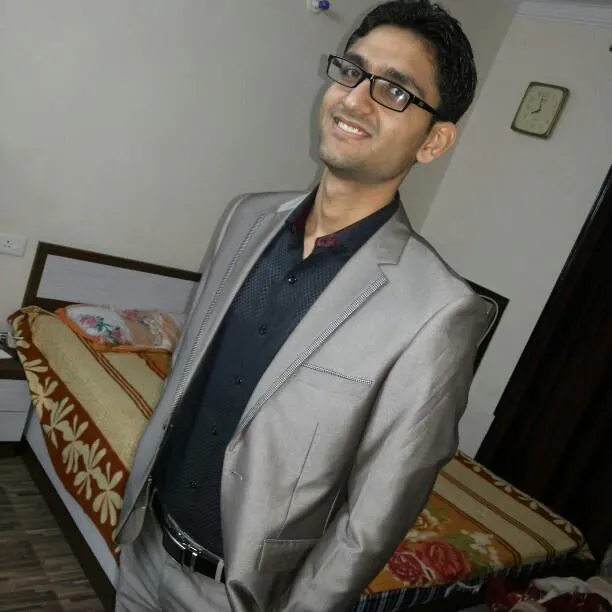# Smart Traffic Lights for EMERGENCY Vehicles

In India there is no option in traffic lights to give way to emergency vehicle which is being implemented in this project

IntermediateFull instructions provided5 days1,793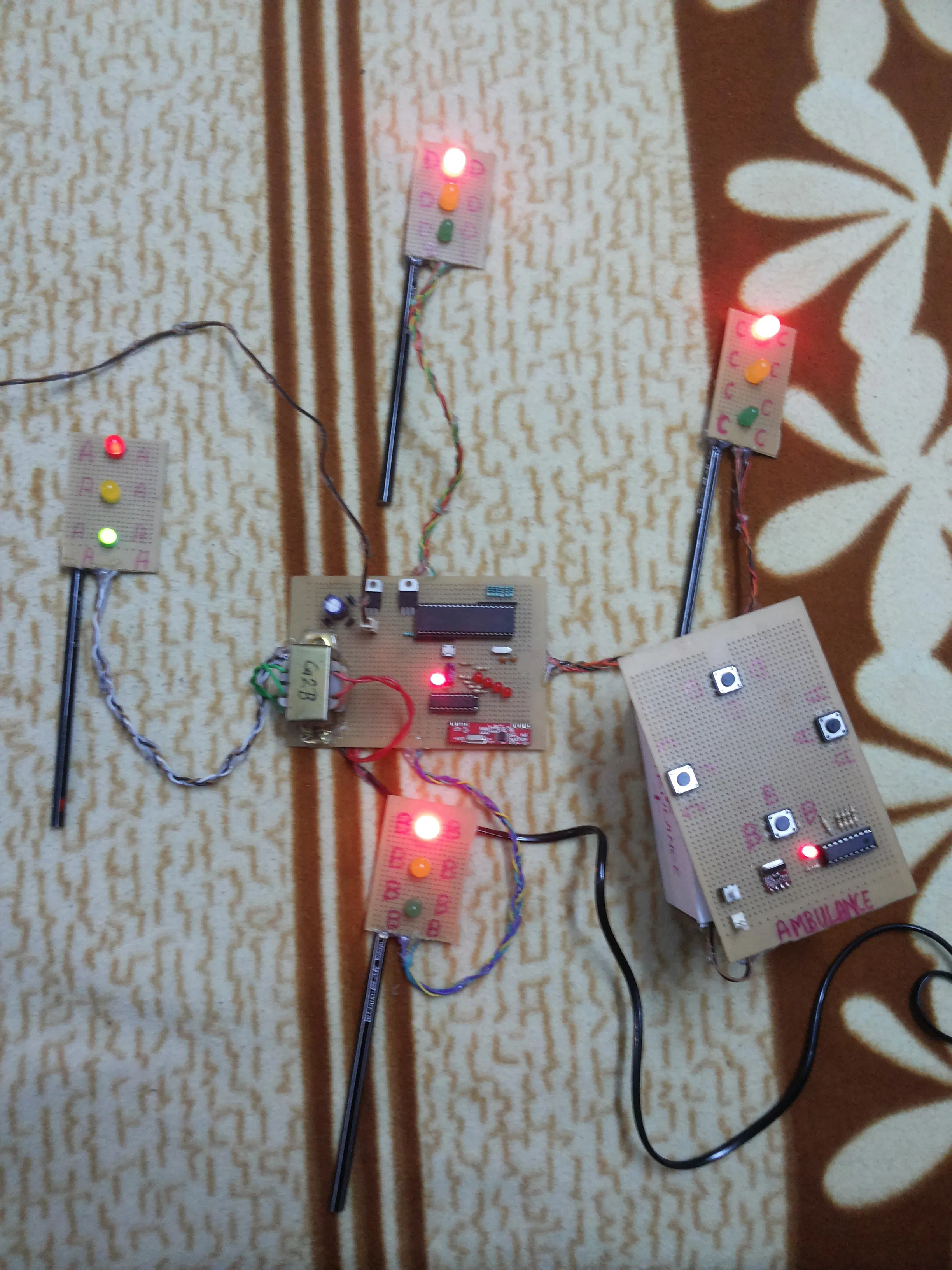## Things used in this project

### Hardware components

 0 9 Transformer
×1
 Diode IN 4007
×1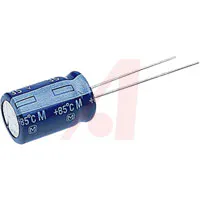Capacitor 1000 µF
×1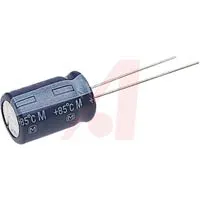Capacitor 10 µF
×1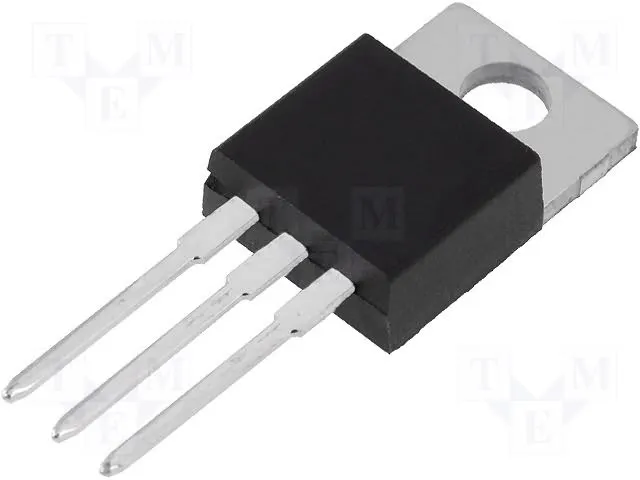Linear Regulator (7805)
×3
 10 mm Red LED
×4
 10 mm Yellow LED
×4
 10 mm Green LED
×2
 Resistance 10 k ohm
×1
 IC AT89C51
×1
 40 pin ic base
×1
 18 pin ic base
×2
 IC HT12D
×1
 IC HT12E
×1
 Tectile Switch (big 4 pin)
×5
 Capacitor 33pf
×2
 Crystal 11.0592 Mhz
×1
 General Purpose PCB (6inch x 4inch)
×3
 Connecting Wire (10 core)
×1
 4.7 K ohm Resistance Sip
×25 mm LED: Red
×6
 Resistance 1.5 K ohm
×10
 Push to ON Switch
×1
 Rechargeable Battery (4.15V)
×1
 Resistance 51 K ohm
×1
 Resistance 1 M ohm
×1
 R F transmitter (4 pin, 434 Mhz)
×1
 R F Receiver (8 pin, 434 Mhz)
×1

### Hand tools and fabrication machinesSoldering iron (generic)

## Schematics

### Traffic Light Section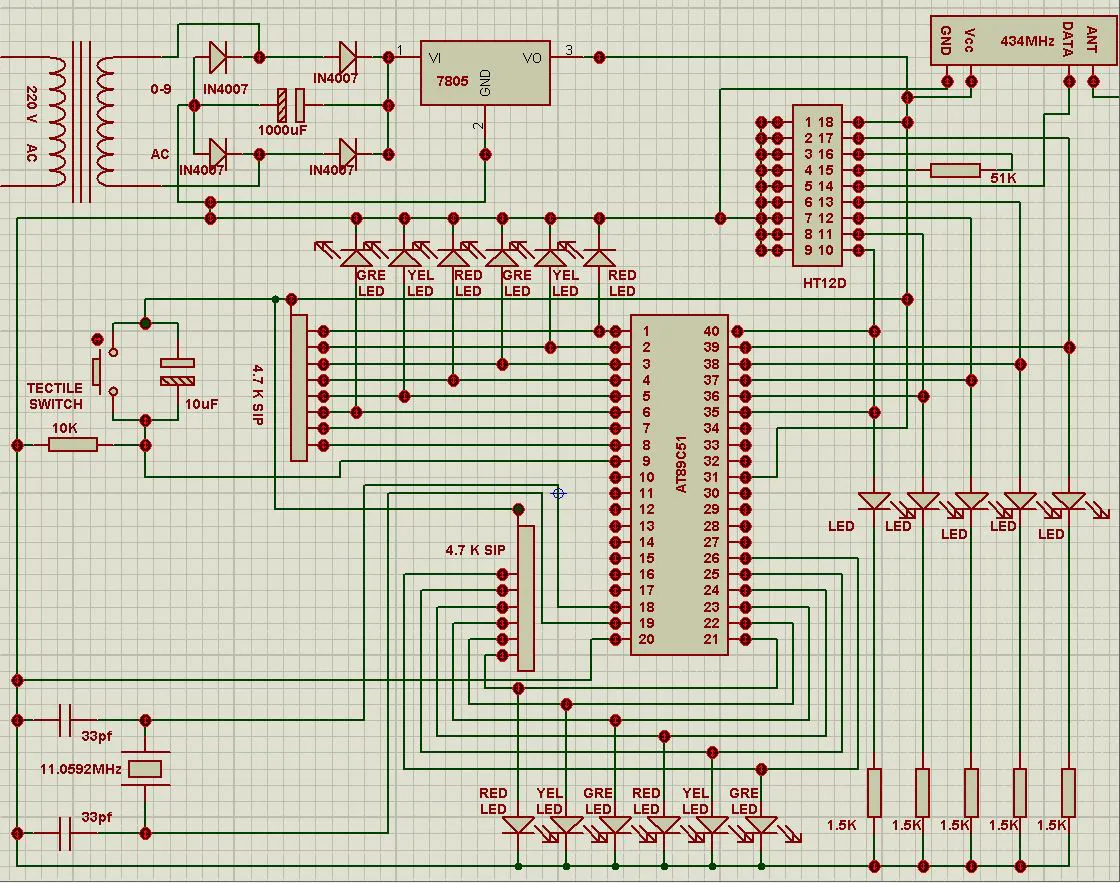### Ambulance or other Emergency Vehicle Section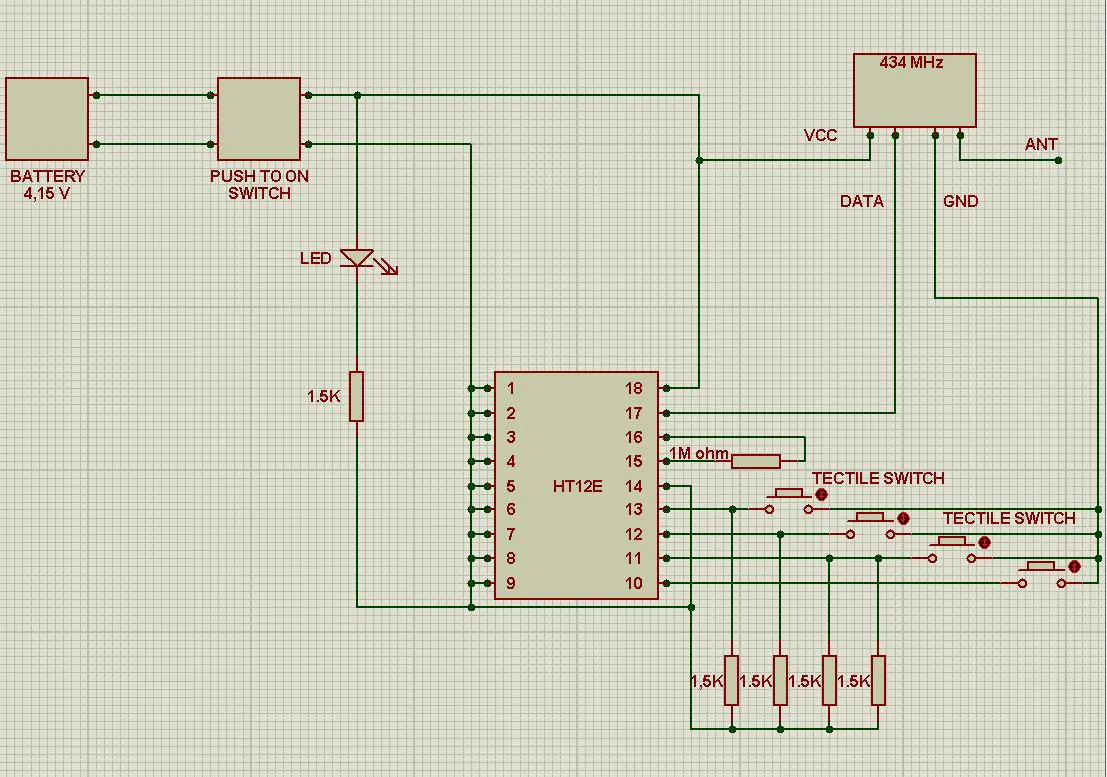## Code

### Smart Traffic Light

C/C++
```#include<reg51.h>

sbit r1=P1^0;
sbit y1=P1^1;
sbit g1=P1^2;
sbit c1=P0^4;

sbit r2=P1^3;
sbit y2=P1^4;
sbit g2=P1^5;
sbit c2=P0^3;

sbit r3=P2^0;
sbit y3=P2^1;
sbit g3=P2^2;
sbit c3=P0^2;

sbit r4=P2^3;
sbit y4=P2^4;
sbit g4=P2^5;
sbit c4=P0^1;

sbit c5=P0^0;

void main()
{
unsigned int a;
unsigned char b,c=0,d=0,e=0;
while(1)
{
qwe:
r1=0;
y1=0;
g1=1;

r2=1;
y2=0;
g2=0;

r3=1;
y3=0;
g3=0;

r4=1;
y4=0;
g4=0;
for(b=0;b<15;b++)
for(a=0;a<40000;a++)
{
if(c5==1)
{
if(c1==1)
goto qwe;
if(c2==1)
{
e=1;
goto wer;
}
if(c3==1)
{
c=1;
goto wer;
}
if(c4==1)
{
d=1;
goto wer;
}
}
}

wer:
r1=0;
y1=1;
g1=0;

r2=1;
y2=0;
g2=0;

r3=1;
y3=0;
g3=0;

r4=1;
y4=0;
g4=0;
if(c==1 || d==1 || e==1)
{
for(a=0;a<40000;a++);
goto mnb;
}
for(b=0;b<2;b++)
for(a=0;a<40000;a++)
{
if(c5==1)
{
if(c1==1)
goto qwe;
if(c2==1)
goto tyu;
if(c3==1)
goto ert;
if(c4==1)
goto rty;
}
}

mnb:
if(c==1)
{
c=0;
goto ert;
}

if(d==1)
{
d=0;
goto rty;
}
e=0;

tyu:
r1=1;
y1=0;
g1=0;

r2=0;
y2=0;
g2=1;

r3=1;
y3=0;
g3=0;

r4=1;
y4=0;
g4=0;
for(b=0;b<15;b++)
for(a=0;a<40000;a++)
{
if(c5==1)
{
if(c1==1)
{
c=1;
goto asd;
}
if(c2==1)
goto tyu;
if(c3==1)
{
e=1;
goto asd;
}
if(c4==1)
{
d=1;
goto asd;
}
}
}

asd:
r1=1;
y1=0;
g1=0;

r2=0;
y2=1;
g2=0;

r3=1;
y3=0;
g3=0;

r4=1;
y4=0;
g4=0;
if(c==1 || d==1 || e==1)
{
for(a=0;a<40000;a++);
goto nbv;
}
for(b=0;b<2;b++)
for(a=0;a<40000;a++)
{
if(c5==1)
{
if(c1==1)
goto qwe;
if(c2==1)
goto tyu;
if(c3==1)
goto ert;
if(c4==1)
goto rty;
}
}

nbv:
if(d==1)
{
d=0;
goto rty;
}

if(c==1)
{
c=0;
goto qwe;
}
e=0;

r1=1;
y1=0;
g1=0;

r2=1;
y2=0;
g2=0;

r3=0;
y3=0;
g3=1;

r4=1;
y4=0;
g4=0;
for(b=0;b<15;b++)
for(a=0;a<40000;a++)
{
if(c5==1)
{
if(c1==1)
{
c=1;
goto zxc;
}
if(c4==1)
{
e=1;
goto zxc;
}
if(c3==1)
goto ert;
if(c2==1)
{
d=1;
goto zxc;
}
}
}

zxc:
r1=1;
y1=0;
g1=0;

r2=1;
y2=0;
g2=0;

r3=0;
y3=1;
g3=0;

r4=1;
y4=0;
g4=0;
if(c==1 || d==1 || e==1)
{
for(a=0;a<40000;a++);
goto bvc;
}
for(b=0;b<2;b++)
for(a=0;a<40000;a++)
{
if(c5==1)
{
if(c1==1)
goto qwe;
if(c2==1)
goto tyu;
if(c3==1)
goto ert;
if(c4==1)
goto rty;
}
}
bvc:
if(d==1)
{
d=0;
goto tyu;
}

if(c==1)
{
c=0;
goto qwe;
}
e=0;

rty:
r1=1;
y1=0;
g1=0;

r2=1;
y2=0;
g2=0;

r3=1;
y3=0;
g3=0;

r4=0;
y4=0;
g4=1;
for(b=0;b<15;b++)
for(a=0;a<40000;a++)
{
if(c5==1)
{
if(c3==1)
{
c=1;
goto xcv;
}
if(c1==1)
{
e=1;
goto xcv;
}
if(c4==1)
goto rty;
if(c2==1)
{
d=1;
goto xcv;
}
}
}
xcv:
r1=1;
y1=0;
g1=0;

r2=1;
y2=0;
g2=0;

r3=1;
y3=0;
g3=0;

r4=0;
y4=1;
g4=0;
if(c==1 || d==1 || e==1)
{
for(a=0;a<40000;a++);
goto vcx;
}
for(b=0;b<2;b++)
for(a=0;a<40000;a++)
{
if(c5==1)
{
if(c1==1)
goto qwe;
if(c2==1)
goto tyu;
if(c3==1)
goto ert;
if(c4==1)
goto rty;
}
}
VCX:
if(d==1)
{
d=0;
goto tyu;
}

if(c==1)
{
c=0;
goto ert;
}
e=0;

}
}
```

## Credits

### Sumit Grover

8 projects • 26 followers